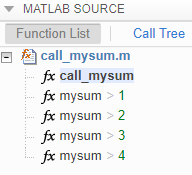## Code Generation for Recursive Functions

To generate code for recursive MATLAB® functions, the code generator uses compile-time recursion or run-time recursion. You can influence whether the code generator uses compile-time or run-time recursion by modifying your MATLAB code. See Force Code Generator to Use Run-Time Recursion.

You can disallow recursion or disable run-time recursion by modifying configuration parameters.

When you use recursive functions in MATLAB code that is intended for code generation, you must adhere to certain restrictions. See Recursive Function Limitations for Code Generation.

### Compile-Time Recursion

With compile-time recursion, the code generator creates multiple versions of a recursive function in the generated code. The inputs to each version have values or sizes that are customized for that version. These versions are known as function specializations. You can see if the code generator used compile-time recursion by looking at the code generation report. Here is an example of compile-time recursion in the report.### Run-Time Recursion

With run-time recursion, the code generator produces a recursive function in the generated code. You can see if the code generator used run-time recursion by looking at the code generation report. Here is an example of run-time recursion in the report.### Disallow Recursion

In a code acceleration configuration object, set the value of the `CompileTimeRecursionLimit` configuration parameter to 0.

### Disable Run-Time Recursion

Some coding standards, such as MISRA®, do not allow recursion. To increase the likelihood of generating code that is compliant with MISRA C®, disable run-time recursion.

In a code acceleration configuration object, set `EnableRuntimeRecursion` to `false`.

If your code requires run-time recursion and run-time recursion is disabled, you must rewrite your code so that it uses compile-time recursion or does not use recursion.

### Recursive Function Limitations for Code Generation

When you use recursion in MATLAB code that is intended for code generation, follow these restrictions:

• Assign all outputs of a run-time recursive function before the first recursive call in the function.

• Assign all elements of cell array outputs of a run-time recursive function.

• Inputs and outputs of run-time recursive functions cannot be classes.

• The `StackUsageMax` code acceleration configuration parameter is ignored for run-time recursion.